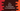# C++ program to check if a character is a hexadecimal using isxdigit## C++ program to check if a character is hexadecimal using isxdigit:

isxdigit is defined in header. This function is used to check if a character is hexadecimal or not. In this post, we will learn how to use isxdigit with examples.

### Definition of isxdigit:

isxdigit is defined as below:

``int isxdigit(int c)``

Here, c is the character to check. It checks as defined by the current C locale.

It returns an integer value. If it is zero, it is false, i.e. not a hexadecimal character. For any other non-zero value, it is true i.e. it is a hexadecimal character.

A hexadecimal digit can be a digit from 0 to 9 or a lowercase character from a to f or uppercase character from A to F.

In the below program, we are taking a character as input from the user and printing the result if it is hexadecimal digit or not.

### C++ program on isxdigit:

Let’s take a look at the below program:

``````#include <iostream>
#include <cctype>

using namespace std;

int main()
{
char ch;

cout<<"Enter a character : "<<endl;
cin>>ch;

if(isxdigit(ch)){
cout<<"It is a hexadecimal digit !"<<endl;
}else{
cout<<"It is not a hexadecimal digit !"<<endl;
}
}``````

Here,

• We are taking one character as input from the user and storing it in ch.
• Using isxdigit, we are checking if it is a hexadecimal digit or not, and based on that, we are printing one message.

It will print output as like below:

``````Enter a character :
x
It is not a hexadecimal digit !

Enter a character :
a
It is a hexadecimal digit !``````